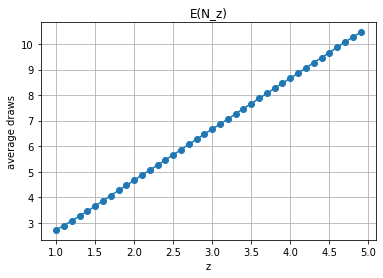# Random numbers, the natural logarithm and higher dimensional simplexes

Marton Trencseni - Sat 17 April 2021 - Data

## Introduction

This is a fun post to exercise our brain and practice college math.

Let's play a game: draw a uniform random number between 0 and 1. What is the expected value?

from random import random

def once(n=1000*1000):
sum = 0.0
for _ in range(n):
sum += random()
return sum/n
print(once())

> 0.50023


As expected, it's 0.5.

Okay, what about if we draw two numbers?

def twice(n=1000*1000):
sum = 0.0
for _ in range(n):
sum += random()
sum += random()
return sum/n
print(twice())

>  1.00003


As expected, it's 1.0.

Okay, now let's turn it on its head: draw random numbers between 0 and 1, and stop when the sum exceeds 1. What is the expected number of draws?

def stop_at(z=1.0, n=1000*1000):
total_draws = 0.0
for _ in range(n):
trial_sum = 0.0
num_draws = 0
while True:
trial_sum += random()
num_draws += 1
if trial_sum >= z: break
total_draws += num_draws
return total_draws/n
print(stop_at(1))

> 2.71758


This returns $e$, the base of the natural logarithm! Why does it show up here?

## Practicing college math

As a reminder, the definition of $e$:

$e = 1 + \dfrac{1}{1!} + \dfrac{1}{2!} + \dfrac{1}{3!} + \dfrac{1}{4!} + ... = 1 + \sum\limits_{n=1}^{\infty} \dfrac{1}{n!}$

Why is this the expected value of the draws?

Let $N$ denote the random variable which denotes the number of draws required to cross 1.0. The expected value:

$E(N) = \sum\limits_{k=2}^{\infty}{ k \times P(N=k)}$

The sum starts at $k = 2$ because we need at least 2 draws, $P(N=k) = 0$ for $k = 1$.

The first step is to realize that $P(N=k)$, the probability that we stop at $k$, is the probability that the sum is less than $1$ at $k-1$ draws, and exceeds $1$ after the $k$-th draw. Let $U_i$ denote the $i$-th draw, and let $S_k$ denote the sum up to $k$, $S_k = \sum\limits_{i=1}^{k}{ U_i }$.

Then:

$P(N=k) = P( S_{k-1} < 1 \land S_k \geq 1 )$

Now some elementary probability theory:

$P(A) = P(A \land B) + P( \land \neg B)$

With $A = S_{k-1} < 1$ and $B = S_{k} \geq 1$:

$P(S_{k-1} < 1) = P( S_{k-1} < 1 \land S_k \geq 1 ) + P( S_{k-1} < 1 \land S_k < 1 )$

Obviously, $P( S_{k-1} < 1 \land S_k < 1 ) = P( S_k < 1 )$, so:

$P(S_{k-1} < 1) = P( S_{k-1} < 1 \land S_k \geq 1 ) + P( S_k < 1 )$

Moving terms:

$P( S_{k-1} < 1 \land S_k \geq 1 ) = P(S_{k-1} < 1) - P( S_k < 1 )$

So:

$P(N=k) = P( S_{k-1} < 1 \land S_k \geq 1 ) = P(S_{k-1} < 1) - P( S_k < 1 )$

Now we just need to figure out the formula for $P( S_k < 1 )$.

This is easiest solved geometrically. For $k=2$, $P( S_k < 1 )$ is the triangle area inside the x-y unit square where $x+y < 1$, which is clearly $1/2$. For $k=3$, $P( S_k < 1 )$ is the simplex volume inside the x-y-z unit square where $x+y+z < 1$. The simplex has as its base the previous triangle defined by $x+y < 1$, and has unit height.The general formula for the area of triangles (volumes of simplexes) with unit height in $k$ dimensions is $V = A \times k$, where A is the area (volume) of the base, which is the same formula for $k-1$. Inductively, this means that:

$P( S_k < 1 ) = \dfrac{1}{k!}$

We have the factorial from the definition of $e$! Now we apply a simple identity of the factorial, $k! = k \times (k-1)!$ to our formula:

$P(N=k) = P(S_{k-1} < 1) - P( S_k < 1 ) = \dfrac{1}{(k-1)!} - \dfrac{1}{k!} = \dfrac{k}{k \times (k-1)!} - \dfrac{1}{k!} = \dfrac{k}{k!} - \dfrac{1}{k!} = \dfrac{k-1}{k!}$

We're almost done:

$E(N) = \sum\limits_{k=2}^{\infty}{ k \times P(N=k)} = \sum\limits_{k=2}^{\infty}{ k \times \dfrac{k-1}{k!} } = \sum\limits_{k=2}^{\infty}{ \dfrac{k-1}{(k-1)!} }$

Let $j = k-1$:

$E(N) = \sum\limits_{j=1}^{\infty}{ \dfrac{j}{j!} } = 1 + \sum\limits_{j=2}^{\infty}{ \dfrac{j}{j!} } = 1 + \sum\limits_{j=2}^{\infty}{ \dfrac{1}{(j-1)!} }$

Let $n = j-1$:

$E(N) = 1 + \sum\limits_{j=2}^{\infty}{ \dfrac{1}{(j-1)!} } = 1 + \sum\limits_{n=1}^{\infty}{ \dfrac{1}{n!} } = e$

## Varying the stopping threshold

We saw above that if we stop at $z=1$, we need an average of $e$ draws. What if we want to stop at $z=2$ or $z=3.14$? What is the $E(N_z)$, the average number of draws required if we want to stop at $z$?

import matplotlib.pyplot as plt

x = [x/10 for x in list(range(10, 50, 1))]
y = [stop_at(i, n=100*1000) for i in x]
fig, ax = plt.subplots()
ax.plot(x, y, marker='o')
ax.set(xlabel='z', ylabel='average draws',
title='E(N_z)')
ax.grid()
plt.show()This is intuitive: if we need $e$ draws to reach $z$, and each additional draws averages $0.5$, so $E(N_z) = f(z) = e + 2(z-1)$.

Sometimes it's good to exercise our brain (and $\LaTeX$ skills) to stay sharp!

The ipython notebook is here.